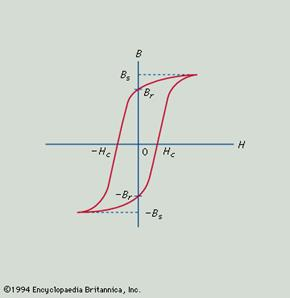# Magnet. Magnetization process. Barium ferrites and Lenz’s law

## MAGNET

It is any material capable of attracting iron and producing a magnetic field outside itself. By the end of the 19th century all the known elements and many compounds had been tested for magnetism, and all were found to have some magnetic property. The most common was the property of diamagnetism, the name given to materials exhibiting a weak repulsion by both poles of a magnet. Some materials, such as chromium, showed paramagnetism, being capable of weak induced magnetization when brought near a magnet. This magnetization disappears when the magnet is removed. Only three elements, iron, nickel, and cobalt, showed the property of ferromagnetism (i.e., the capability of remaining permanently magnetized).Magnetization process.

The quantities now used in characterizing magnetization were defined and named by William Thomson (Lord Kelvin) in 1850. The symbol B denotes the magnitude of magnetic flux density inside a magnetized body, and the symbol H denotes the magnitude of magnetizing force, or magnetic field, producing it. The two are represented by the equation B = mH, in which the Greek letter mu, m, symbolizes the permeability of the material and is a measure of the intensity of magnetization that can be produced in it by a given magnetic field. The modern units of the International Standard (SI) system for B are teslas (T) or webers per square metre (Wb/m2) and for H are amperes per metre (A/m). The units were formerly called, respectively, gauss and oersted. The units of m are henrys per metre.All ferromagnetic materials exhibit the phenomenon of hysteresis, a lag in response to changing forces based on energy losses resulting from internal friction. If B is measured for various values of H and the results are plotted in graphic form, the result is a loop of the type shown in the accompanying figure, called a hysteresis loop. The name describes the situation in which the path followed by the values of B while H is increasing differs from that followed as H is decreasing. With the aid of this diagram, the characteristics needed to describe the performance of a material to be used as a magnet can be defined. Bs is the saturation flux density and is a measure of how strongly the material can be magnetized. Br is the remanent flux density and is the residual, permanent magnetization left after the magnetizing field is removed; this latter is obviously a measure of quality for a permanent magnet. It is usually measured in webers per square metre. In order to demagnetize the specimen from its remanent state, it is necessary to apply a reversed magnetizing field, opposing the magnetization in the specimen. The magnitude of field necessary to reduce the magnetization to zero is Hc, the coercive force, measured in amperes per metre. For a permanent magnet to retain its magnetization without loss over a long period of time, Hc should be as large as possible. The combination of large Br and large Hc will generally be found in a material with a large saturation flux density that requires a large field to magnetize it. Thus, permanent-magnet materials are often characterized by quoting

Тип:
Конспекты лекций
Размер файла:
407 Kb
Скачали:
0